# ISBLANK in ExcelArticle byExcelMojo Team## What Is ISBLANK Function In Excel?

The ISBLANK in Excel detects the blank cells (empty cells) and returns the answer in Boolean. So, if any blank cells are detected, we get the result TRUE; else, we get FALSE. The ISBLANK in Excel was introduced in the year 2007.

For example, consider the below table with values in column A. Now, we need to find the blank cells using ISBLANK in Excel. The steps used to find blank cells using ISBLANK in Excel are:

First, we should select the cell where we want to display the result. So, in this example, select cell B2. Next, enter the ISBLANK formula in cell B2. So, the complete formula is =ISBLANK(A2). Press Enter key. Clearly, we can see that the function has returned FALSE for the value in cell A2 and TRUE for cell A3.

Thus, we can find blank cells in Excel using ISBLANK in excel.

###### Key Takeaways
• The ISBLANK function in Excel is a logical function that checks whether the searched cell is blank or not.
• It is a single argument function and was introduced in the year 2007.
• The formula is =ISBLANK(value), where value is a mandatory argument that denotes the target cell which we want to test.
• We can access the function by selecting Formulas > More Functions > Information > ISBLANK.
• Even though a cell looks empty, it may not be blank if there is a space in the cell. That is why the ISBLANK function is used in Excel.

### ISBLANK() Excel Formula

The formula of ISBLANK in Excel is –

where, value is a mandatory argument and denotes the target cell we want to test.

### How To Use ISBLANK Excel Function? (With Steps)

There are two methods to insert ISBLANK in excel function in our worksheets. They are:

1.Access from the Excel ribbon

• First, choose an empty cell to display the result.
• Next, go to the Formulas tab and select More Functions from the Function Library group.
• Then, click on Information and select the ISBLANK option from the drop-down menu.
• The Function Arguments window appears.
• Then, enter the value in the Value box depending on the number of arguments.
• Select OK

2. Enter the worksheet manually

• Select an empty cell to view the result.
• Type =ISBLANK( in the selected cell.

Alternatively, type =I and double-click on the ISBLANK function from the list of suggestions shown by Excel.

#Basic Example

The following table shows values in column A. Now, we need to use the below steps to find blank cells in excel using ISBLANK.

The steps to detect blank cells using ISBLANK in Excel are as follows:

1. First, select the column where we will enter the formula and calculate the result.

So, the selected column, in this case, is column B.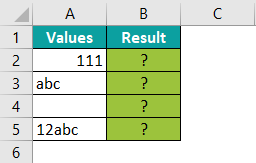2. Next, enter the ISBLANK formula in cell B2.

So, the complete formula is =ISBLANK(A2). We can see that the value argument is cell A2.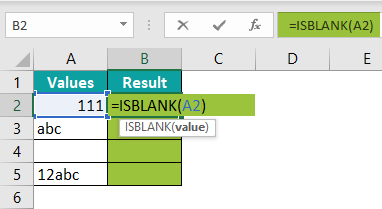3. Press Enter key.

The calculated result is FALSE in cell A2.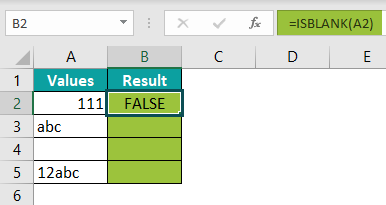4. Now, using the AutoFill option, drag the cursor to cell B5.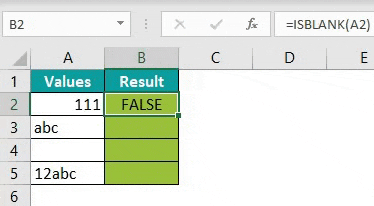The obtained results show that the table has only one blank cell, i.e., cell A4.
Likewise, we can find blank cells in excel using ISBLANK in excel function.

### Examples

#### Example – 1 – Using Conditional Formatting

The below table has some values distributed randomly. Therefore, we need to use the following steps in excel to find blank cells with ISBLANK in Excel using conditional formatting.

The steps to detect blank cells with ISBLANK in Excel are as follows:

Step 1: To begin with, select the table array.

Step 2: Next, go to the Home tab.

Step 3: Then, select the Conditional Formatting option from the Styles group.

Step 4: Now, choose the New Rule… option from the drop-down list.

Step 5: The New Formatting Rule window pops up.

Step 6: Next, select the Use a formula to determine which cells to format option.

Step 7: Then, enter the formula in the Format values where this formula is true: box.

So, the formula is =ISBLANK(A2:F4).

Step 8: Click the Format… button to set the color of the blank cells.

Step 9: The Format Cells window appears.

Step 10: Next, in the Fill menu option, select the color from the Background Color: section.

Step 11: Click OK.

Step 12: The Format Cells window gets closed, and a new window called the New Formatting Rule pops up.

Step 13: Click OK.

Step 14: Now, we can see that the blank cells are highlighted in green, as shown in the below image.

Similarly, we can easily detect the blank cells using ISBLANK and conditional formatting.

#### Example – 2 – Using IF function

The following table has values in column A. First, we identify the blank cells with the ISBLANK in Excel using the IF function.

The steps to detect the blank cells with IF in Excel and ISBLANK are:

Step 1: First, select the cell in which we enter the ISBLANK formula.

So, in our example, the selected cell is B2.

Step 2: Next, enter the ISBLANK formula with the IF Excel formula in cell B2.

So, instead of returning ‘TRUE’ or ‘FALSE’ values, the functions return ‘Cell is Empty’ and ‘Cell is not empty’ in this example.

Step 3: Therefore, the complete formula is,

=IF(ISBLANK(A2),“Cell is Empty”,“Cell is not Empty”).

Step 4: Press Enter key.

Clearly, the function has returned the value, ‘Cell is Empty’ in cell B2, as shown in the above image.

Step 5: Now, drag the cursor using the AutoFill option till cell B5. Excel automatically returns the output, as shown in the image below.

Clearly, we can see that the cells B2 and B4 are blank because the function has returned the value, ‘Cell is Empty,’ whereas the other cells have values (non-blank cells) and is indicated by ‘Cell is not empty’ value.

Similarly, we can find the blank and non-blank cells with IF ISBLANK in Excel.

#### Example – 3 – Using VLOOKUP function

The below table shows the contact details of people. First, we need to find the blank cells using the VLOOKUP in Excel and ISBLANK.

In the table,

• Column A displays the names of people
• Column B shows the contact No.
• Column C contains the email IDs.

The steps to find the blank cells with ISBLANK in Excel are:

Step 1: To begin with, select the cell to display the output.

Step 2: Next, enter the ISBLANK formula with IF and VLOOKUP formulas in cell F2.

So, the complete formula is:

Step 3: Press Enter key.

Step 4: The result is obtained as ‘Received’ in cell F2, as shown in the following image.

Step 5: Now, drag the cursor to cell F5 to obtain results in other cells.

Clearly, the table shows that cells F2 and F4 show ‘Received’ values, and cells C2 and C4 show ‘Not Received’ values. From the output, we can understand that cells C3 and C5 are blank.

Likewise, we can use VLOOKUP in Excel and ISBLANK to obtain the results.

### Things to Remember

• The ISBLANK function in Excel is used to find blank cells (empty cells) in the worksheet.
• It can only be applied to a single cell at a time; it cannot be used for a table range.
• This function can also be used with other functions like IF, VLOOKUP, etc.
• The ISBLANK function in Excel can be used to do conditional formatting.
• It is easy to find blank cells within a large data set.
• The ISEMPTY function is a VBA function.

ISBLANK is Not Working in Excel?

The ISBLANK function in Excel returns TRUE for empty cells and FALSE for non-blank cells. But sometimes, it may not work in excel. So, let us understand the reasons with an example.

Consider the table with values in column A. Now, we need to find the blank cells in excel.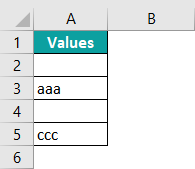The steps used to find blank cells in excel are as follows:

Step 1: First, select the cell where we want to display the result.
So, in this case, it is B2.

Step 2: Next, enter the formula in cell B2.

So, the complete formula is =ISBLANK(A2)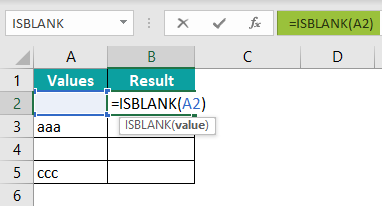Step 3: Then, press the Enter key.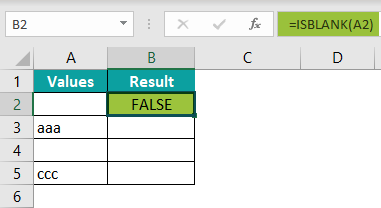Though cell A2 looks empty without any visible content, the function has returned FALSE because there is space in the cell.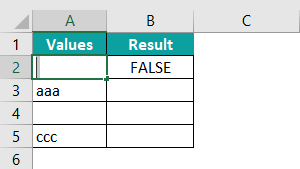Similarly, the function may not work properly.

So, we must check the following:
1. Check for extra spaces in-between formulas.
2. Treat 0-length strings as blank cells.
3. Make sure the cells do not have any spaces.

How to write an ISBLANK formula in Excel?

The steps used to write the ISBLANK formula in Excel are;

1. First, select the cell.
2. Next, type the formula =ISBLANK(
3. Then, select the value for arguments.
4. Press ENTER.

So, the complete formula is =ISBLANK(value).

When to use ISBLANK in Excel?

The ISBLANK function in Excel is an information function used to find non-empty cells, i.e., the blank cells. The function returns TRUE if the data has a blank cell and returns FALSE if the data has non-blank cells.

In simple terms, the ISBLANK function checks a specified cell and identifies whether it is blank or not.